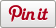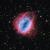# Vectors in 3 Dimensions

•• Sharebar
•## Vectors in 3 Dimensions

### Mathematical background

In mathematics a vector has a magnitude (length) and direction expressed as an ordered list of tuples (x, y, z). It is sketched as a directed line segment (arrow). Unless otherwise given, a vector does not impart information about location (when studying in secondary education, we use the origin (0,0,0) of a set of axis as the initial point of a vector.

Unit vectors

in the direction of the x-axis

j in the direction of the y-axis

k in the direction of the z-axis

a vector is written in the form ai + bj + ck

where a, b and c represent the distance from the origin (0,0,0)

hence 2i  + 3j -  1k would be

• 2 units along the x-axis – this can be achieved using the command EAST
• 3 units up the y-axis– this can be achieved using the command UP
• 1 unit forward along the z-axis– this can be achieved using the command SOUTH

To show the vector 2i +3j -1k

we need to move east 2, up 3 and north -1

to show the final vector only we can set the final position – MAKE “A POS

LINE PD SETPOS “A PU

Full code

File: basic_vector.x3d

note: use the command LABEL POS to show the current position

We can then make this into a more generic code

We can now create any vector

This is a very simplistic way to show our vector. As we explore more complex questions we need to alter our processes.